# (1 point) A steel ball weighing 128 pounds is suspended from a spring. This stretches the...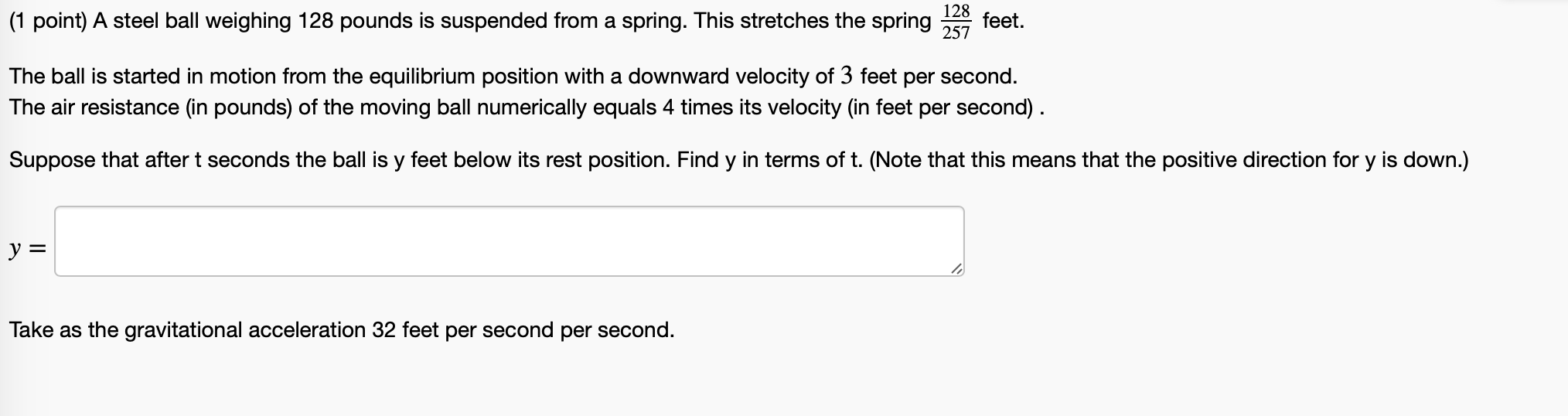(1 point) A steel ball weighing 128 pounds is suspended from a spring. This stretches the spring 128 257 feet. The ball is started in motion from the equilibrium position with a downward velocity of 3 feet per second. The air resistance (in pounds) of the moving ball numerically equals 4 times its velocity (in feet per second). Suppose that after t seconds the ball is y feet below its rest position. Find y in terms of t. (Note that this means that the positive direction for y is down.) y = Take as the gravitational acceleration 32 feet per second per second.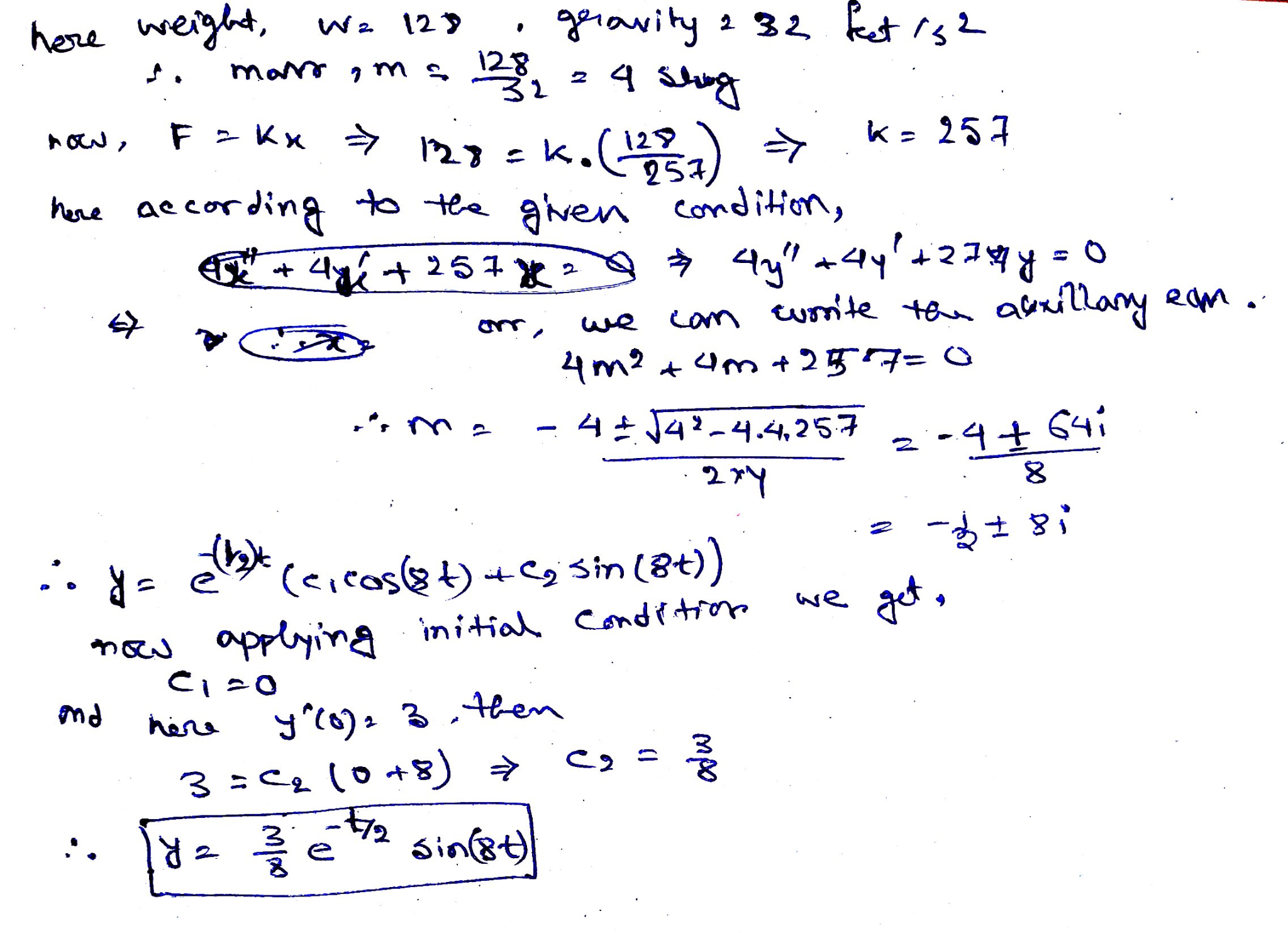Thank you so much sir

##### Add Answer of: (1 point) A steel ball weighing 128 pounds is suspended from a spring. This stretches the...
Similar Homework Help Questions
• ### (1 point) A steel ball weighing 128 pounds is suspended from a spring. This stretches the...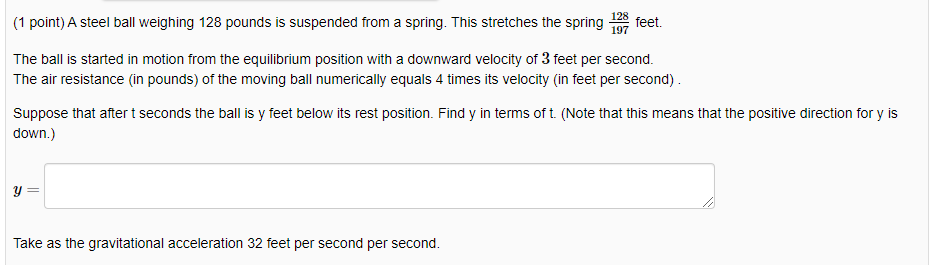(1 point) A steel ball weighing 128 pounds is suspended from a spring. This stretches the spring feet. The ball is started in motion from the equilibrium position with a downward velocity of 3 feet per second. The air resistance (in pounds) of the moving ball numerically equals 4 times its velocity (in feet per second). Suppose that after t seconds the ball is y feet below its rest position. Find y in terms of t. (Note that this means...

• ### (1 point) A steel ball weighing 128 pounds is suspended from a spring This stretches the...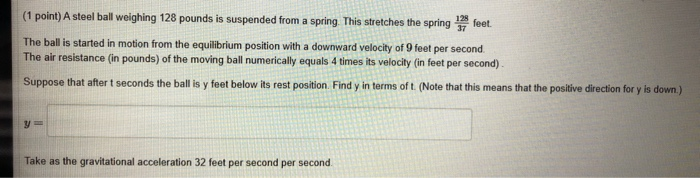(1 point) A steel ball weighing 128 pounds is suspended from a spring This stretches the spring 13 feet The ball is started in motion from the equilibrium position with a downward velocity of 9 feet per second The air resistance (in pounds) of the moving ball numerically equals 4 times its velocity (in feet per second) Suppose that after t seconds the ball is y feet below its rest position Find y in terms of t. (Note that this...

• ### 145 (1 point) A steel ball weighing 128 pounds is suspended from a spring. This stretches...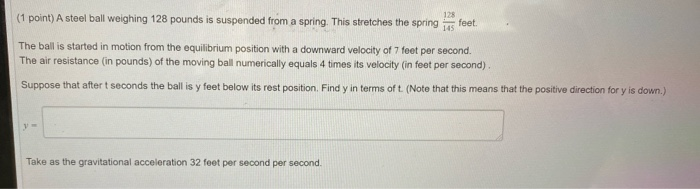145 (1 point) A steel ball weighing 128 pounds is suspended from a spring. This stretches the spring 128 feet The ball is started in motion from the equilibrium position with a downward velocity of 7 feet per second. The air resistance (in pounds) of the moving ball numerically equals 4 times its velocity (in feet per second). Suppose that after t seconds the ball is y feet below its rest position. Find y in terms of t. (Note that...

• ### Problem 1. 128 325 feet. (1 point) A steel ball weighing 128 pounds is suspended from...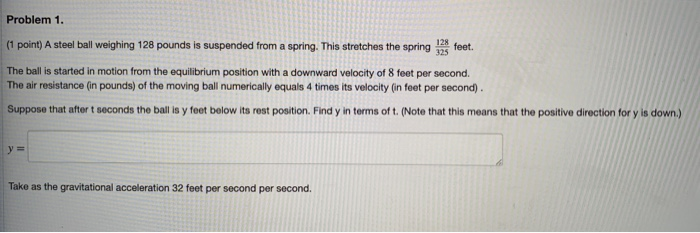Problem 1. 128 325 feet. (1 point) A steel ball weighing 128 pounds is suspended from a spring. This stretches the spring The ball is started in motion from the equilibrium position with a downward velocity of 8 feet per second. The air resistance (in pounds) of the moving ball numerically equals 4 times its velocity (in feet per second) Suppose that after t seconds the ball is y feet below its rost position. Find y in terms of t....

• ### Problem 1. (point) A steel ball weighing 128 pounds is suspended from a spring. This stretches...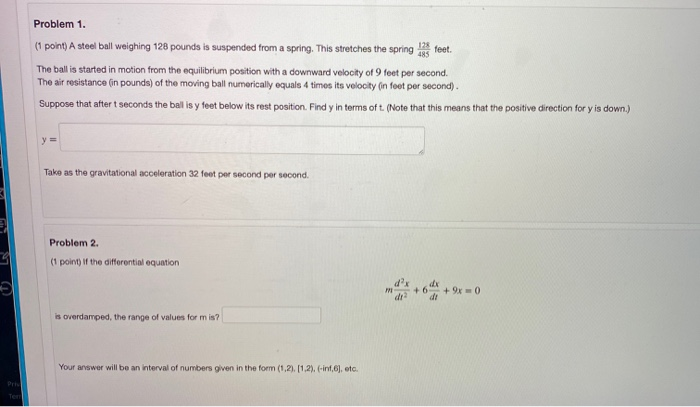Problem 1. (point) A steel ball weighing 128 pounds is suspended from a spring. This stretches the spring feet. The ball is started in motion from the equilibrium position with a downward velocity of 9 feet per second. The air resistance (in pounds) of the moving ball numerically equals 4 times its velocity on feet per second). Suppose that after t seconds the ball is y feet below its rest position. Find y in terms of t. (Note that this...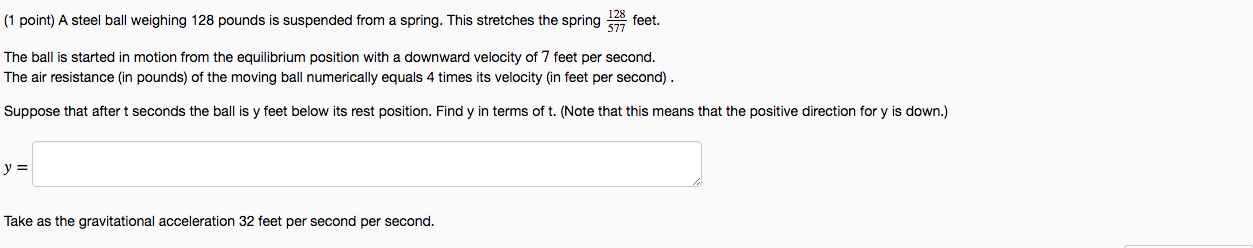PLEASE ANSWER BOTH PROBLEM SETS PLEASE ANSWER BOTH (1 point) A steel ball weighing 128 pounds is suspended from a spring. This stretches the spring 528 feet. The ball is started in motion from the equilibrium position with a downward velocity of 7 feet per second. The air resistance (in pounds) of the moving ball numerically equals 4 times its velocity (in feet per second). Suppose that after t seconds the ball is y feet below its rest position. Find...

• ### A hollow steel ball weighing 24 pounds is suspended from a spring.

A hollow steel ball weighing 24 pounds is suspended from a spring. This stretches the spring 4 inches. The ball is started in motion from a point 3 inches above theequilibrium position.Let u(t) be the displacement of the mass from equilibrium. Suppose that after t seconds the ball is u feet below its rest position. Find u (in feet) in terms of t.(Note that the positive direction is down.)Take as the gravitational acceleration 32 feet per second per second.

• ### DiffEQ: Mechanical Vibrations: a steel ball weighing 128 pounds suspended from a spring.(1 pt) A steel ball weighing 128 pounds is suspended from a spring. This stretches the spring feet.The ball is started in motion from the equilibrium position with a downward velocity of 2 feet per second.The air resistance (in pounds) of the moving ball numerically equals 4 times its velocity (in feet per second) .Suppose that after t seconds the ball is y feet below its rest position. Find y in terms of t. (Note that this means that the...

• ### spring

A hollow steel ball weighing 4 pounds is suspended from a spring. This stretches the spring feet. The ball is started in motion from the equilibrium position with adownward velocity of feet per second. The air resistance (in pounds) of the moving ball numerically equals 4 times its velocity (in feet per second) . Suppose thatafter t seconds the ball is y feet below its rest position. Find y in terms of t.

• ### Defined that natural length is unknown, can we assume its zero? do we need natural length?...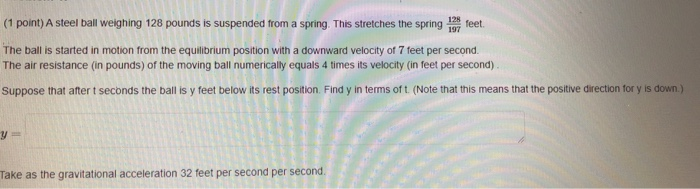Defined that natural length is unknown, can we assume its zero? do we need natural length? 128 feet 197 (1 point) A steel ball weighing 128 pounds is suspended from a spring. This stretches the spring The ball is started in motion from the equilibrium position with a downward velocity of 7 feet per second. The air resistance (in pounds) of the moving ball numerically equals 4 times its velocity (in feet per second) Suppose that after seconds the ballis...

Free Homework App